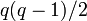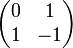# Element structure of general linear group:GL(2,3)

## Contents

View element structure of particular groups | View other specific information about general linear group:GL(2,3)

This article describes the element structure of general linear group:GL(2,3) which is the general linear group of degree two over field:F3.

## Conjugacy class structure

### Interpretation as general linear group of degree two over field:F3

Nature of conjugacy class Eigenvalues Characteristic polynomial Minimal polynomial Size of conjugacy class (generic$q$) Size of conjugacy class ($q = 3$) Number of such conjugacy classes (generic$q$) Number of such conjugacy classes ($q = 3$) Total number of elements (generic$q$) Total number of elements ($q = 3$) Representative matrix (one per conjugacy class)
Diagonalizable over$\mathbb{F}_3$ with equal diagonal entries, hence a scalar$\{a,a \}$ where$a \in \mathbb{F}_3^\ast$$(x - a)^2$ where$a \in \mathbb{F}_3^\ast$$x - a$ where$a \in \mathbb{F}_3^\ast$ 1 1$q - 1$ 2$q - 1$ 2$\begin{pmatrix}1 & 0 \\ 0 & 1 \\\end{pmatrix}$,$\begin{pmatrix}-1 & 0 \\ 0 & -1 \\\end{pmatrix}$
Diagonalizable over field:F9, not over$\mathbb{F}_3$. Must necessarily have no repeated eigenvalues. Pair of conjugate elements of$\mathbb{F}_{9}$$x^2 + 1$,$x^2 + x - 1$,$x^2 - x - 1$ Same as characteristic polynomial$q(q - 1)$ 6$q(q-1)/2$ 3$q^2(q-1)^2/2$ 18$\begin{pmatrix} 0 & 1 \\ -1 & 0 \\\end{pmatrix}$,$\begin{pmatrix} 0 & 1 \\ 1 & -1 \\\end{pmatrix}$,$\begin{pmatrix} 0 & 1 \\ 1 & 1 \\\end{pmatrix}$
Not diagonal, has Jordan block of size two$a$ (multiplicity two) where$a \in \mathbb{F}_3^\ast$$(x - a)^2$ where$a \in \mathbb{F}_3^\ast$ Same as characteristic polynomial$q^2 - 1$ 8$q - 1$ 2$(q + 1)(q - 1)^2$ 16$\begin{pmatrix} 1 & 1 \\ 0 & 1 \\\end{pmatrix}$,$\begin{pmatrix} -1 & 1 \\ 0 & -1 \\\end{pmatrix}$
Diagonalizable over field:F3 with distinct diagonal entries$\{ 1, -1 \}$$x^2 - 1$ Same as characteristic polynomial$q(q + 1)$ 12$(q - 1)(q - 2)/2$ 1$q(q+1)(q-1)(q-2)/2$ 12$\begin{pmatrix} 1 & 0 \\ 0 & -1 \\\end{pmatrix}$
Total NA NA NA NA NA$q^2 - 1$ 8$q^4 - q^3 - q^2 + q$ 48 NA